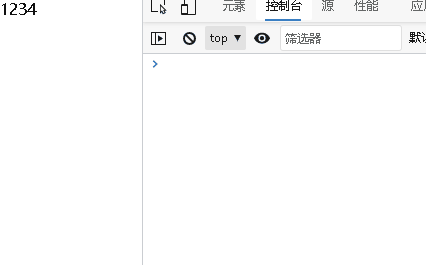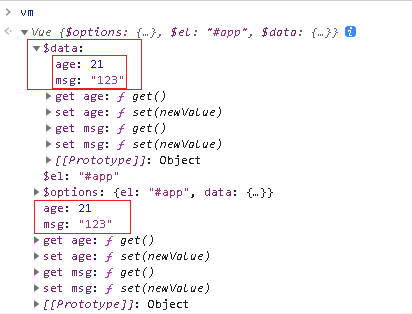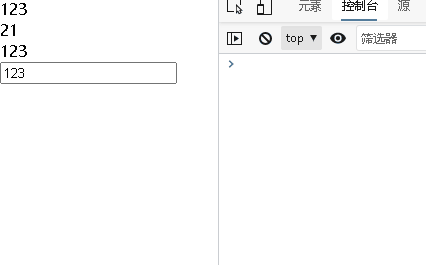# Vue2.X响应式原理

## 1.defineProperty 的应用

Vue2.X 响应式中使用到了 defineProperty 进行数据劫持，所以我们对它必须有一定的了解，那么我们先来了解它的使用方法把， 这里我们来使用 defineProperty来模拟 Vue 中的 data

``````<body>
<div id="app"></div>
<script>
// 模拟 Vue的data
let data = {
msg: '',
}
// 模拟 Vue 实例
let vm = {}
// 对 vm 的 msg 进行数据劫持
Object.defineProperty(vm, 'msg', {
// 获取数据
get() {
return data.msg
},
// 设置 msg
set(newValue) {
// 如果传入的值相等就不用修改
if (newValue === data.msg) return
// 修改数据
data.msg = newValue
document.querySelector('#app').textContent = data.msg
},
})
// 这样子就调用了 defineProperty vm.msg 的 set
vm.msg = '1234'
</script>
</body>
``````## 2.defineProperty修改多个参数为响应式

``````<body>
<div id="app"></div>
<script>
// 模拟 Vue的data
let data = {
msg: '哈哈',
age: '18',
}
// 模拟 Vue 实例
let vm = {}
// 把多个属性转化 响应式
function proxyData() {
// 把data 中每一项都[msg,age] 拿出来操作
Object.keys(data).forEach((key) => {
// 对 vm 的 属性 进行数据劫持
Object.defineProperty(vm, key, {
// 可枚举
enumerable: true,
// 可配置
configurable: true,
// 获取数据
get() {
return data[key]
},
// 设置 属性值
set(newValue) {
// 如果传入的值相等就不用修改
if (newValue === data[key]) return
// 修改数据
data[key] = newValue
document.querySelector('#app').textContent = data[key]
},
})
})
}
// 调用方法
proxyData(data)

</script>
</body>
``````

## 3.Proxy

Vue3 中使用 Proxy 来设置响应式的属性

`new Proxy(target,handler)`

• `target` ：要使用 `Proxy` 包装的目标对象（可以是任何类型的对象，包括原生数组，函数，甚至另一个代理）
• `handler`：一个通常以函数作为属性的对象，各属性中的函数分别定义了在执行各种操作时代理 `p` 的行为

``````<body>
<div id="app"></div>
<script>
// 模拟 Vue data
let data = {
msg: '',
age: '',
}
// 模拟 Vue 的一个实例
// Proxy 第一个
let vm = new Proxy(data, {
// get() 获取值
// target 表示需要代理的对象这里指的就是 data
// key 就是对象的 键
get(target, key) {
return target[key]
},
// 设置值
// newValue 是设置的值
set(target, key, newValue) {
// 也先判断下是否和之前的值一样 节省性能
if (target[key] === newValue) return
// 进行设置值
target[key] = newValue
document.querySelector('#app').textContent = target[key]
},
})
</script>
</body>
``````

``````// 触发了set方法
vm.msg = 'haha'
// 触发了get方法
console.log(vm.msg)
``````

## 4.发布订阅模式

``````<body>
<div id="app"></div>
<script>
class Vue {
constructor() {
// 用来存储事件
// 存储的 例子 this.subs = { 'myclick': [fn1, fn2, fn3] ,'inputchange': [fn1, fn2] }
this.subs = {}
}
// 实现 \$on 方法 type是任务队列的类型 ,fn是方法
\$on(type, fn) {
// 判断在 subs是否有当前类型的 方法队列存在
if (!this.subs[type]) {
// 没有就新增一个 默认为空数组
this.subs[type] = []
}
// 把方法加到该类型中
this.subs[type].push(fn)
}
// 实现 \$emit 方法
\$emit(type) {
// 首先得判断该方法是否存在
if (this.subs[type]) {
// 获取到参数
const args = Array.prototype.slice.call(arguments, 1)
// 循环队列调用 fn
this.subs[type].forEach((fn) => fn(...args))
}
}
}

// 使用
const eventHub = new Vue()
// 使用 \$on 添加一个 sum 类型的 方法到 subs['sum']中
eventHub.\$on('sum', function () {
let count = [...arguments].reduce((x, y) => x + y)
console.log(count)
})
// 触发 sum 方法
eventHub.\$emit('sum', 1, 2, 4, 5, 6, 7, 8, 9, 10)
</script>
</body>
``````

## 5.观察者模式

``````<body>
<div id="app"></div>
<script>
// 目标
class Subject {
constructor() {
this.observerLists = []
}
// 添加观察者
// 判断观察者是否有 和 存在更新订阅的方法
if (obs && obs.update) {
// 添加到观察者列表中
this.observerLists.push(obs)
}
}
// 通知观察者
notify() {
this.observerLists.forEach((obs) => {
// 每个观察者收到通知后 会更新事件
obs.update()
})
}
// 清空观察者
empty() {
this.subs = []
}
}

class Observer {
// 定义观察者内容更新事件
update() {
// 在更新事件要处理的逻辑
console.log('目标更新了')
}
}

// 使用
// 创建目标
let sub = new Subject()
// 创建观察者
let obs1 = new Observer()
let obs2 = new Observer()
// 把观察者添加到列表中
// 目标开启了通知 每个观察者者都会自己触发 update 更新事件
sub.notify()
</script>
</body>
``````

## 6.模拟Vue的响应式原理

• 接收初始化的参数，这里只举几个简单的例子 el data options
• 通过私有方法 _proxyDatadata 注册到 Vue 中 转成getter setter
• 使用 observerdata 中的属性转为 响应式 添加到 自身身上
• 使用 observer 方法监听 data 的所有属性变化来 通过观察者模式 更新视图
• 使用 compiler 编译元素节点上面指令 和 文本节点差值表达式

### 1.vue.js

``````/* vue.js */

class Vue {
constructor(options) {
// 获取到传入的对象 没有默认为空对象
this.\$options = options || {}
// 获取 el
this.\$el =
typeof options.el === 'string'
? document.querySelector(options.el)
: options.el
// 获取 data
this.\$data = options.data || {}
// 调用 _proxyData 处理 data中的属性
this._proxyData(this.\$data)
}
// 把data 中的属性注册到 Vue
_proxyData(data) {
Object.keys(data).forEach((key) => {
// 进行数据劫持
// 把每个data的属性 到添加到 Vue 转化为 getter setter方法
Object.defineProperty(this, key, {
// 设置可以枚举
enumerable: true,
// 设置可以配置
configurable: true,
// 获取数据
get() {
return data[key]
},
// 设置数据
set(newValue) {
// 判断新值和旧值是否相等
if (newValue === data[key]) return
// 设置新值
data[key] = newValue
},
})
})
}
}
``````

### 2.observer.js

``````/* observer.js */

class Observer {
constructor(data) {
// 用来遍历 data
this.walk(data)
}
// 遍历 data 转为响应式
walk(data) {
// 判断 data是否为空 和 对象
if (!data || typeof data !== 'object') return
// 遍历 data
Object.keys(data).forEach((key) => {
// 转为响应式
this.defineReactive(data, key, data[key])
})
}
// 转为响应式
// 要注意的 和vue.js 写的不同的是
// vue.js中是将 属性给了 Vue 转为 getter setter
// 这里是 将data中的属性转为getter setter
defineReactive(obj, key, value) {
// 如果是对象类型的 也调用walk 变成响应式，不是对象类型的直接在walk会被return
this.walk(value)
// 保存一下 this
const self = this
Object.defineProperty(obj, key, {
// 设置可枚举
enumerable: true,
// 设置可配置
configurable: true,
// 获取值
get() {
return value
},
// 设置值
set(newValue) {
// 判断旧值和新值是否相等
if (newValue === value) return
// 设置新值
value = newValue
// 赋值的话如果是newValue是对象，对象里面的属性也应该设置为响应式的
self.walk(newValue)
},
})
}
}

``````

``````<script src="./js/observer.js"></script>
<script src="./js/vue.js"></script>
``````

``````/* vue.js */

class Vue {
constructor(options) {
...
// 使用 Obsever 把data中的数据转为响应式
new Observer(this.\$data)
}
// 把data 中的属性注册到 Vue
_proxyData(data) {
...
}
}

``````

obsever.js 中是把 data 的所有属性 加到 data 自身 变为响应式 转成 getter setter方式

vue.js 中 也把 data的 的所有属性 加到 Vue 上,是为了以后方面操作可以用 Vue 的实例直接访问到 或者在 Vue 中使用 this 访问

``````<body>
<div id="app"></div>
<script src="./js/observer.js"></script>
<script src="./js/vue.js"></script>
<script>
let vm = new Vue({
el: '#app',
data: {
msg: '123',
age: 21,
},
})
</script>
</body>
``````### 3.compiler.js

comilper.js在这个文件里实现对文本节点 和 元素节点指令编译 主要是为了举例子 当然这个写的很简单 指令主要实现 v-text v-model

``````/* compiler.js */

class Compiler {
// vm 指 Vue 实例
constructor(vm) {
// 拿到 vm
this.vm = vm
// 拿到 el
this.el = vm.\$el
// 编译模板
this.compile(this.el)
}
// 编译模板
compile(el) {
// 获取子节点 如果使用 forEach遍历就把伪数组转为真的数组
let childNodes = [...el.childNodes]
childNodes.forEach((node) => {
// 根据不同的节点类型进行编译
// 文本类型的节点
if (this.isTextNode(node)) {
// 编译文本节点
this.compileText(node)
} else if (this.isElementNode(node)) {
//元素节点
this.compileElement(node)
}
// 判断是否还存在子节点考虑递归
if (node.childNodes && node.childNodes.length) {
// 继续递归编译模板
this.compile(node)
}
})
}
// 编译文本节点(简单的实现)
compileText(node) {
// 核心思想利用把正则表达式把{{}}去掉找到里面的变量
// 再去Vue找这个变量赋值给node.textContent
let reg = /\{\{(.+?)\}\}/
// 获取节点的文本内容
let val = node.textContent
// 判断是否有 {{}}
if (reg.test(val)) {
// 获取分组一  也就是 {{}} 里面的内容 去除前后空格
let key = RegExp.\$1.trim()
// 进行替换再赋值给node
node.textContent = val.replace(reg, this.vm[key])
}
}
// 编译元素节点这里只处理指令
compileElement(node) {
// 获取到元素节点上面的所有属性进行遍历
![...node.attributes].forEach((attr) => {
// 获取属性名
let attrName = attr.name
// 判断是否是 v- 开头的指令
if (this.isDirective(attrName)) {
// 除去 v- 方便操作
attrName = attrName.substr(2)
// 获取 指令的值就是  v-text = "msg"  中msg
// msg 作为 key 去Vue 找这个变量
let key = attr.value
// 指令操作 执行指令方法
// vue指令很多为了避免大量个 if判断这里就写个 uapdate 方法
this.update(node, key, attrName)
}
})
}
// 添加指令方法 并且执行
update(node, key, attrName) {
// 比如添加 textUpdater 就是用来处理 v-text 方法
// 我们应该就内置一个 textUpdater 方法进行调用
// 加个后缀加什么无所谓但是要定义相应的方法
let updateFn = this[attrName + 'Updater']
// 如果存在这个内置方法 就可以调用了
updateFn && updateFn(node, key, this.vm[key])
}
// 提前写好 相应的指定方法比如这个 v-text
// 使用的时候 和 Vue 的一样
textUpdater(node, key, value) {
node.textContent = value
}

// v-model
modelUpdater(node, key, value) {
node.value = value
}

// 判断元素的属性是否是 vue 指令
isDirective(attr) {
return attr.startsWith('v-')
}
// 判断是否是元素节点
isElementNode(node) {
return node.nodeType === 1
}
// 判断是否是 文本 节点
isTextNode(node) {
return node.nodeType === 3
}
}

``````

### 4.dep.js

``````/* dep.js */

class Dep {
constructor() {
// 存储观察者
this.subs = []
}
// 添加观察者
// 判断观察者是否存在 和 是否拥有update方法
if (sub && sub.update) {
this.subs.push(sub)
}
}
// 通知方法
notify() {
// 触发每个观察者的更新方法
this.subs.forEach((sub) => {
sub.update()
})
}
}

``````

obsever.js 中使用Dep

get 中添加 Dep.target (观察者)

set 中 触发 notify (通知)

``````/* observer.js */

class Observer {
...
}
// 遍历 data 转为响应式
walk(data) {
...
}
// 这里是 将data中的属性转为getter setter
defineReactive(obj, key, value) {
...
// 创建 Dep 对象
let dep = new Dep()
Object.defineProperty(obj, key, {
...
// 获取值
get() {
// 在这里添加观察者对象 Dep.target 表示观察者
return value
},
// 设置值
set(newValue) {
if (newValue === value) return
value = newValue
self.walk(newValue)
// 触发通知 更新视图
dep.notify()
},
})
}
}

``````

### 5.watcher.js

**watcher **的作用 数据更新后 收到通知之后 调用 update 进行更新

``````/* watcher.js */

class Watcher {
constructor(vm, key, cb) {
// vm 是 Vue 实例
this.vm = vm
// key 是 data 中的属性
this.key = key
// cb 回调函数 更新视图的具体方法
this.cb = cb
// 把观察者的存放在 Dep.target
Dep.target = this
// 旧数据 更新视图的时候要进行比较
// 还有一点就是 vm[key] 这个时候就触发了 get 方法
// 之前在 get 把 观察者 通过dep.addSub(Dep.target) 添加到了 dep.subs中
this.oldValue = vm[key]
// Dep.target 就不用存在了 因为上面的操作已经存好了
Dep.target = null
}
// 观察者中的必备方法 用来更新视图
update() {
// 获取新值
let newValue = this.vm[this.key]
// 比较旧值和新值
if (newValue === this.oldValue) return
// 调用具体的更新方法
this.cb(newValue)
}
}

``````

``````/* compiler.js */

class Compiler {
// vm 指 Vue 实例
constructor(vm) {
// 拿到 vm
this.vm = vm
// 拿到 el
this.el = vm.\$el
// 编译模板
this.compile(this.el)
}
// 编译模板
compile(el) {
let childNodes = [...el.childNodes]
childNodes.forEach((node) => {
if (this.isTextNode(node)) {
// 编译文本节点
this.compileText(node)
}
...
}
// 编译文本节点(简单的实现)
compileText(node) {
let reg = /\{\{(.+)\}\}/
let val = node.textContent
if (reg.test(val)) {
let key = RegExp.\$1.trim()
node.textContent = val.replace(reg, this.vm[key])
// 创建观察者
new Watcher(this.vm, key, newValue => {
node.textContent = newValue
})
}
}
...
// v-text
textUpdater(node, key, value) {
node.textContent = value
// 创建观察者2
new Watcher(this.vm, key, (newValue) => {
node.textContent = newValue
})
}
// v-model
modelUpdater(node, key, value) {
node.value = value
// 创建观察者
new Watcher(this.vm, key, (newValue) => {
node.value = newValue
})
// 这里实现双向绑定 监听input 事件修改 data中的属性
this.vm[key] = node.value
})
}
}

``````

``````new Watcher(this.vm, key, newValue => {
node.textContent = newValue
})
``````

## 7.测试下自己写的

``````<body>
<div id="app">
{{msg}} <br />
{{age}} <br />
<div v-text="msg"></div>
<input v-model="msg" type="text" />
</div>
<script src="./js/dep.js"></script>
<script src="./js/watcher.js"></script>
<script src="./js/compiler.js"></script>
<script src="./js/observer.js"></script>
<script src="./js/vue.js"></script>
<script>
let vm = new Vue({
el: '#app',
data: {
msg: '123',
age: 21,
},
})
</script>
</body>
``````OK 基本实现了 通过 观察者模式 来 实现 响应式原理

## 8.五个文件代码

vue.js

``````/* vue.js */

class Vue {
constructor(options) {
// 获取到传入的对象 没有默认为空对象
this.\$options = options || {}
// 获取 el
this.\$el =
typeof options.el === 'string'
? document.querySelector(options.el)
: options.el
// 获取 data
this.\$data = options.data || {}
// 调用 _proxyData 处理 data中的属性
this._proxyData(this.\$data)
// 使用 Obsever 把data中的数据转为响应式
new Observer(this.\$data)
// 编译模板
new Compiler(this)
}
// 把data 中的属性注册到 Vue
_proxyData(data) {
Object.keys(data).forEach((key) => {
// 进行数据劫持
// 把每个data的属性 到添加到 Vue 转化为 getter setter方法
Object.defineProperty(this, key, {
// 设置可以枚举
enumerable: true,
// 设置可以配置
configurable: true,
// 获取数据
get() {
return data[key]
},
// 设置数据
set(newValue) {
// 判断新值和旧值是否相等
if (newValue === data[key]) return
// 设置新值
data[key] = newValue
},
})
})
}
}

``````

obsever.js

``````/* observer.js */

class Observer {
constructor(data) {
// 用来遍历 data
this.walk(data)
}
// 遍历 data 转为响应式
walk(data) {
// 判断 data是否为空 和 对象
if (!data || typeof data !== 'object') return
// 遍历 data
Object.keys(data).forEach((key) => {
// 转为响应式
this.defineReactive(data, key, data[key])
})
}
// 转为响应式
// 要注意的 和vue.js 写的不同的是
// vue.js中是将 属性给了 Vue 转为 getter setter
// 这里是 将data中的属性转为getter setter
defineReactive(obj, key, value) {
// 如果是对象类型的 也调用walk 变成响应式，不是对象类型的直接在walk会被return
this.walk(value)
// 保存一下 this
const self = this
// 创建 Dep 对象
let dep = new Dep()
Object.defineProperty(obj, key, {
// 设置可枚举
enumerable: true,
// 设置可配置
configurable: true,

// 获取值
get() {
// 在这里添加观察者对象 Dep.target 表示观察者
return value
},
// 设置值
set(newValue) {
// 判断旧值和新值是否相等
if (newValue === value) return
// 设置新值
value = newValue
// 赋值的话如果是newValue是对象，对象里面的属性也应该设置为响应式的
self.walk(newValue)
// 触发通知 更新视图
dep.notify()
},
})
}
}

``````

compiler.js

``````/* compiler.js */

class Compiler {
// vm 指 Vue 实例
constructor(vm) {
// 拿到 vm
this.vm = vm
// 拿到 el
this.el = vm.\$el
// 编译模板
this.compile(this.el)
}
// 编译模板
compile(el) {
// 获取子节点 如果使用 forEach遍历就把伪数组转为真的数组
let childNodes = [...el.childNodes]
childNodes.forEach((node) => {
// 根据不同的节点类型进行编译
// 文本类型的节点
if (this.isTextNode(node)) {
// 编译文本节点
this.compileText(node)
} else if (this.isElementNode(node)) {
//元素节点
this.compileElement(node)
}
// 判断是否还存在子节点考虑递归
if (node.childNodes && node.childNodes.length) {
// 继续递归编译模板
this.compile(node)
}
})
}
// 编译文本节点(简单的实现)
compileText(node) {
// 核心思想利用把正则表达式把{{}}去掉找到里面的变量
// 再去Vue找这个变量赋值给node.textContent
let reg = /\{\{(.+?)\}\}/
// 获取节点的文本内容
let val = node.textContent
// 判断是否有 {{}}
if (reg.test(val)) {
// 获取分组一  也就是 {{}} 里面的内容 去除前后空格
let key = RegExp.\$1.trim()
// 进行替换再赋值给node
node.textContent = val.replace(reg, this.vm[key])
// 创建观察者
new Watcher(this.vm, key, (newValue) => {
node.textContent = newValue
})
}
}
// 编译元素节点这里只处理指令
compileElement(node) {
// 获取到元素节点上面的所有属性进行遍历
![...node.attributes].forEach((attr) => {
// 获取属性名
let attrName = attr.name
// 判断是否是 v- 开头的指令
if (this.isDirective(attrName)) {
// 除去 v- 方便操作
attrName = attrName.substr(2)
// 获取 指令的值就是  v-text = "msg"  中msg
// msg 作为 key 去Vue 找这个变量
let key = attr.value
// 指令操作 执行指令方法
// vue指令很多为了避免大量个 if判断这里就写个 uapdate 方法
this.update(node, key, attrName)
}
})
}
// 添加指令方法 并且执行
update(node, key, attrName) {
// 比如添加 textUpdater 就是用来处理 v-text 方法
// 我们应该就内置一个 textUpdater 方法进行调用
// 加个后缀加什么无所谓但是要定义相应的方法
let updateFn = this[attrName + 'Updater']
// 如果存在这个内置方法 就可以调用了
updateFn && updateFn.call(this, node, key, this.vm[key])
}
// 提前写好 相应的指定方法比如这个 v-text
// 使用的时候 和 Vue 的一样
textUpdater(node, key, value) {
node.textContent = value
// 创建观察者
new Watcher(this.vm, key, (newValue) => {
node.textContent = newValue
})
}
// v-model
modelUpdater(node, key, value) {
node.value = value
// 创建观察者
new Watcher(this.vm, key, (newValue) => {
node.value = newValue
})
// 这里实现双向绑定
this.vm[key] = node.value
})
}

// 判断元素的属性是否是 vue 指令
isDirective(attr) {
return attr.startsWith('v-')
}
// 判断是否是元素节点
isElementNode(node) {
return node.nodeType === 1
}
// 判断是否是 文本 节点
isTextNode(node) {
return node.nodeType === 3
}
}

``````

dep.js

``````/* dep.js */

class Dep {
constructor() {
// 存储观察者
this.subs = []
}
// 添加观察者
// 判断观察者是否存在 和 是否拥有update方法
if (sub && sub.update) {
this.subs.push(sub)
}
}
// 通知方法
notify() {
// 触发每个观察者的更新方法
this.subs.forEach((sub) => {
sub.update()
})
}
}

``````

watcher.js

``````/* watcher.js */

class Watcher {
constructor(vm, key, cb) {
// vm 是 Vue 实例
this.vm = vm
// key 是 data 中的属性
this.key = key
// cb 回调函数 更新视图的具体方法
this.cb = cb
// 把观察者的存放在 Dep.target
Dep.target = this
// 旧数据 更新视图的时候要进行比较
// 还有一点就是 vm[key] 这个时候就触发了 get 方法
// 之前在 get 把 观察者 通过dep.addSub(Dep.target) 添加到了 dep.subs中
this.oldValue = vm[key]
// Dep.target 就不用存在了 因为上面的操作已经存好了
Dep.target = null
}
// 观察者中的必备方法 用来更新视图
update() {
// 获取新值
let newValue = this.vm[this.key]
// 比较旧值和新值
if (newValue === this.oldValue) return
// 调用具体的更新方法
this.cb(newValue)
}
}

``````